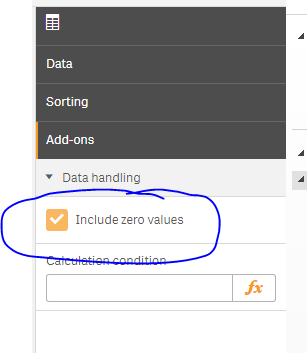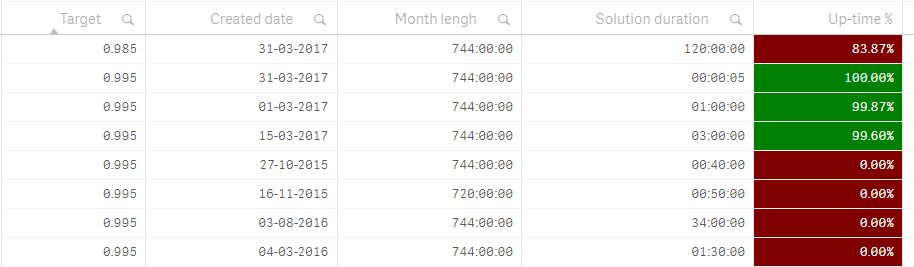# Qlik Sense App Development

Discussion board where members can learn more about Qlik Sense App Development and Usage.

HighlightedPartner

## IF() overruling Set Analysis?

Hi all,

I'm in doubt if I'm doing something wrong.

I have a table with dimensions and measures. On the measure I have a Set Analysis that limits the date range to last three months. All is working fine, no problem, data is only shown for the last three months.

Then I add a cell color condition with a IF-statement and then all rows are shown. It's like the Set Analysis is overrulled by the IF statement.

What I'm I doing wrong?

Br Thomas

Tags (2)
1 Solution

Accepted SolutionsMVP

## Re: IF() overruling Set Analysis?

May be uncheck 'Include zero values' under Add-Ons -> Data handling9 Replies
Contributor III

## Re: IF() overruling Set Analysis?

Hi Thomas,

If you are using the same expression in the color condition you must add the same set analysis in order to keep the same data shown.

Carlos MPartner

## Re: IF() overruling Set Analysis?

Hi carlosamonroy‌,

Okay, but how would you construct a calculation that uses Set Analysis and chooses a color depending on comparison?

Br ThomasMVP

## Re: IF() overruling Set Analysis?

Would you be able to share the expression you are using right now?

Contributor III

## Re: IF() overruling Set Analysis?

The same way you are doing it in the expression.

Measure:

sum({<Field1={'abc'},Field2={'def'}>}Value)

Color condition:

if(sum({<Field1={'abc'},Field2={'def'}>}Value) > 90,RGB(255,0,0),RGB(0,255,0))Partner

## Re: IF() overruling Set Analysis?

Thanks carlosamonroyPartner

## Re: IF() overruling Set Analysis?

carlosamonroy‌, I stand corrected, it doesn't workHere's the code:

gvCurrentMonthM3FirstDate = 01-03-2017

gvLastMonthLastDate = 31-05-2017

Measure

=Sum({\$<[Created date]={'>=\$(gvCurrentMonthM3FirstDate) <=\$(gvLastMonthLastDate)'}>}([Month lengh]-[Solution duration])/[Month lengh])

Text condition

If(Sum({\$<[Created date]={'>=\$(gvCurrentMonthM3FirstDate) <=\$(gvLastMonthLastDate)'}>}([Month lengh]-[Solution duration])/[Month lengh])<Target, Red(),Green())

The last four lines should not be shown.MVP

## Re: IF() overruling Set Analysis?

May be uncheck 'Include zero values' under Add-Ons -> Data handlingContributor III

## Re: IF() overruling Set Analysis?

Can you try using the following expression:

=Sum({\$<[Created date]={'>=\$(gvCurrentMonthM3FirstDate) <=\$(gvLastMonthLastDate)'}>}[Month lengh]-[Solution duration])

/

Sum({\$<[Created date]={'>=\$(gvCurrentMonthM3FirstDate) <=\$(gvLastMonthLastDate)'}>}[Month lengh])Partner

## Re: IF() overruling Set Analysis?

That's the one. Thx stalwar1